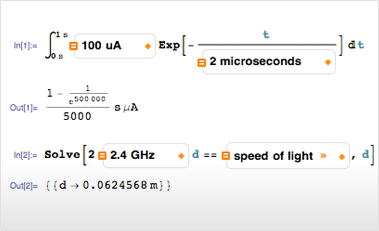# Units

Mathematica 9 introduces a new unit system containing thousands of different units, all integrated with Wolfram|Alpha's sophisticated unit interpretation system. From unit conversion to dimensional analysis, Mathematica provides you with all the tools you need to work with, and extract properties from, units and quantities. Mathematica also includes integration for Quantity in system functions, including numeric and symbolic calculations, statistics, and visualizations.• Convert between different units and unit systems, including complete support for SI, imperial, and metric unit systems. »
• Integration with symbolic and numerical functions including Solve, Integrate, FindRoot, and many more. »
• Get Quantity expressions straight from Wolfram|Alpha. »
• Use units directly in visualization functions.
• Use units in statistics functions. »
• Seamless computation of units involving currency. »
• Robust algorithms to handle conversions and arithmetic using different temperature units. »

## Mathematica

Questions? Comments? Contact a Wolfram expert »